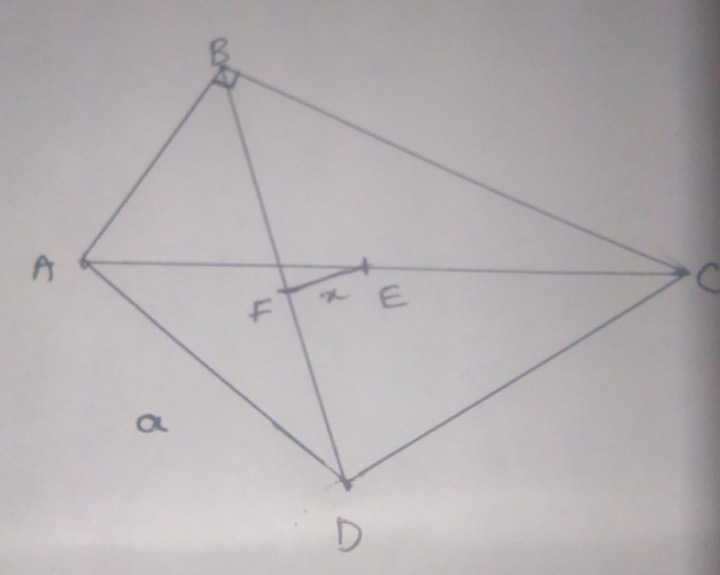# Confusing Triangles

Geometry Level 2In the following figure, ABC is a right angled triangle at B. BDC is an equilateral triangle, E and F are the midpoints of AC and BD respectively, then x=

×